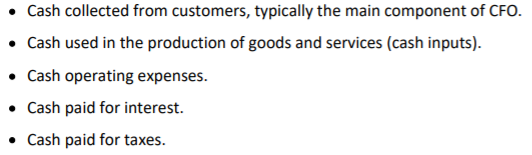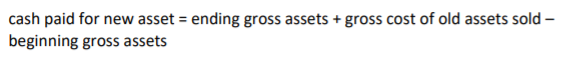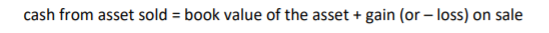# Calculation of CFO, CFF and CFI

Some points to remember

• CFO is calculated differently but the result is the same under both methods
• CFI and CFF is identical under both methods
• Inverse relationship between asset and cash
• An asset account increase = use of cash
• An asset decrease = source of cash
• Direct relationship between liability and cash flow
• Increase of liability account = source of cash
• Decrease in liability = use of cash
• Source of cash are positive number (cash inflows) and use of cash are negative numbers (Cash outflows)

Direct method

• Provide information about where cash comes from and went out
• CFO ‘s common components
•• CFI is calculated by examining the change in the gross asset accounts that result from investing activities
• Fixed asset (48)
• It is necessary to consider if old assets are sold:
•• It is necessary to consider any gain or loss from the sale
•• Intangible asset (49)
• Investment securities (50)
• CFF measures CF occuring between the firm and its suppliers of capital
• Between the firm and its creditor
• Positive CFF: new borrowing, negative CFF: debt principal repayments
• Between the firm and its shareholders
• Positive CFF: equity issued, negative CFF: shares are repurchased, dividends are paid
•• Total cash flow = CFO + CFI + CFF

Indirect method

• CFO
• Begin with net income
• Adjust for differences between accounting items and actual cash receipts and cash disbursements
• Add/subtract non-cash components of revenue: depreciation
• Subtract gains/plus loss on the disposal of assets
• Proceeds from sales of fixed assets are investing cash flow
• Accounts receivable increased → need to subtract this in net income (not received yet)
• Accounts payable increased → Need to add this in net income (not paid yet)
• Calculate CFO under indirect method
• Step 1: begin with net income
• Step 2: Subtract gain or loss that resulted from financing or investing
• Step 3: Add back all noncash charges to income and subtract all noncash components of revenue
• Step 4: Add or subtract changes to balance sheet operating accounts